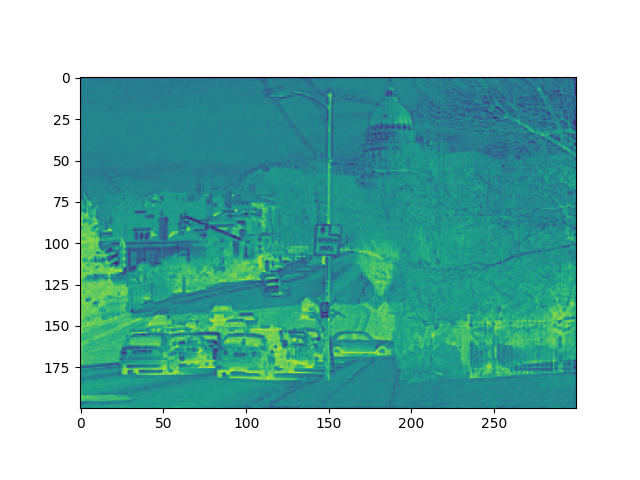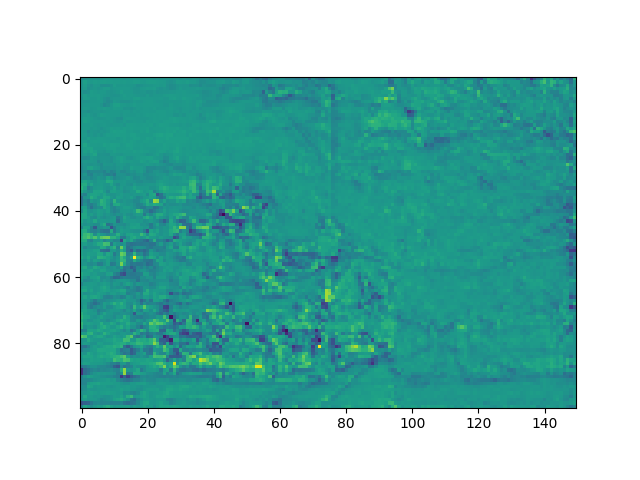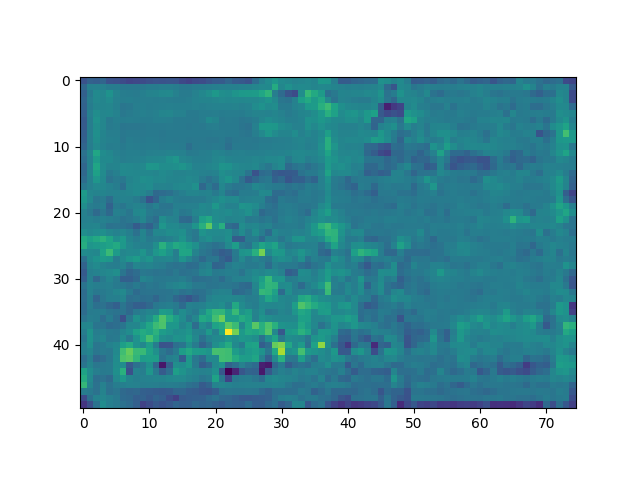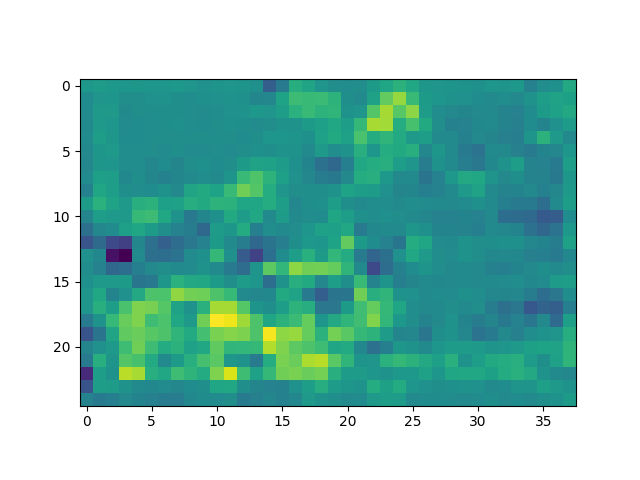## 神经网络输出中间特征图

### 原图：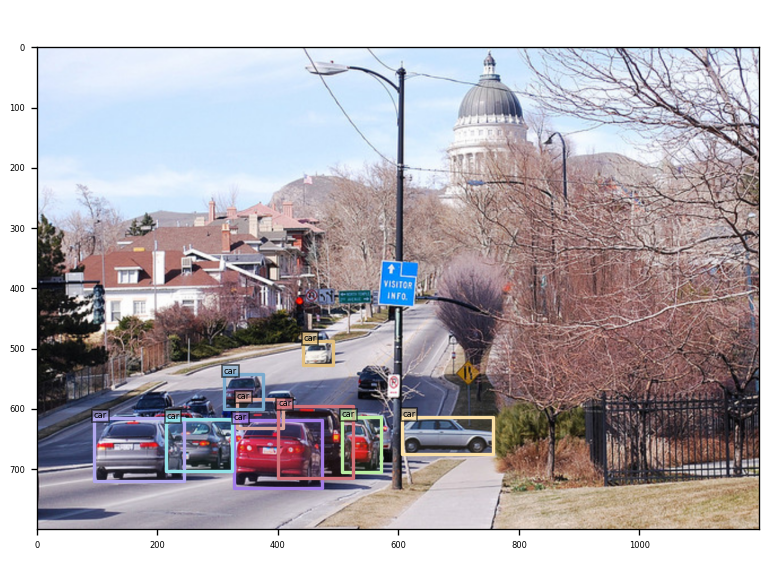### 输出的特征图：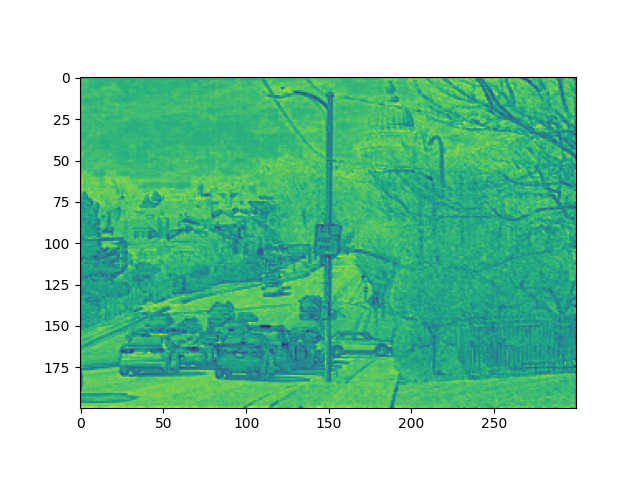``````x的shape: torch.Size([1, 64, 200, 300])
x的shape: torch.Size([1, 128, 100, 150])
x的shape: torch.Size([1, 320, 50, 75])
x的shape: torch.Size([1, 512, 25, 38])
``````

## 代码实现

``````sb = x.cpu().data.numpy()
np.save('matric'+str(i)+'.npy', sb)#这里的i是对应四个阶段的id
``````

``````import numpy as np
import os
import matplotlib.pyplot as plt
import torch
import torch.nn as nn

def normalization(data):  # NORMALIZE TO [0,1]
_range = np.max(data) - np.min(data)
data = (data - np.min(data)) / _range  # [0,1]
return data

def fm_vis(feats, save_dir, save_name):
save_dir = os.path.join(save_dir, save_name)
if not os.path.exists(save_dir):
os.makedirs(save_dir)

feats = normalization(feats.cpu().data.numpy())
for idx in range(min(feats.shape, 200*300)):  # CHANNLE NUMBER
fms = feats[idx, :, :]
plt.imshow(fms)
plt.savefig(os.path.join(save_dir, save_name + '_' + str(idx) + ".png"))

for i in range(0,4):
print(s_b1)
s_b2 = torch.from_numpy(s_b1)
out_dir = "outputs"
s_b = s_b2.reshape(1, 64, 200, 300)
fm_vis(s_b, out_dir, "s_b_vis"+str(i))
``````